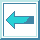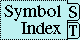#Special Operator LOCALLY

Syntax:

locally declaration* form* => result*

Arguments and Values:

Declaration---a declare expression; not evaluated.

forms---an implicit progn.

results---the values of the forms.

Description:

Sequentially evaluates a body of forms in a lexical environment where the given declarations have effect.

Examples:

``` (defun sample-function (y)  ;this y is regarded as special
(declare (special y))
(let ((y t))              ;this y is regarded as lexical
(list y
(locally (declare (special y))
;; this next y is regarded as special
y))))
=>  SAMPLE-FUNCTION
(sample-function nil) =>  (T NIL)
(setq x '(1 2 3) y '(4 . 5)) =>  (4 . 5)

;;; The following declarations are not notably useful in specific.
;;; They just offer a sample of valid declaration syntax using LOCALLY.
(locally (declare (inline floor) (notinline car cdr))
(declare (optimize space))
(floor (car x) (cdr y))) =>  0, 1
```

```;;; This example shows a definition of a function that has a particular set
;;; of OPTIMIZE settings made locally to that definition.
(locally (declare (optimize (safety 3) (space 3) (speed 0)))
(defun frob (w x y &optional (z (foo x y)))
(mumble x y z w)))
=>  FROB

;;; This is like the previous example, except that the optimize settings
;;; remain in effect for subsequent definitions in the same compilation unit.
(declaim (optimize (safety 3) (space 3) (speed 0)))
(defun frob (w x y &optional (z (foo x y)))
(mumble x y z w))
=>  FROB
```

Side Effects: None.

Affected By: None.

Exceptional Situations: None.